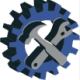## Types of loads – Strength of MaterialWe all know, every machine in this world is built for the purpose of doing some particular work. For doing any type of work, one or many types of forces are transferred from many parts of the machine. Hence, while designing any particular part of machine, one should know all types of forces/ loads coming or acting on that particular part.

A load can be defined as the combination of one or more forces acting on the body. SI unit of the load is ‘Newton’. There are many ways, we can classify the types of loads; but for calculation of various types of stresses and strains in ‘strength of materials’, the types of loads can be-

1. Axial load/ Direct load- When the axis of the body and the line of action of the load coincides with each other then it is called as ‘axial or direct load’. This load always passes through the center of gravity of all cross-sections of the body and acts.

Axial load can be further classified into-

i) Tensile load- When axial load acting on the body is of pull type and the body is subjected to tension, then it is called as tensile load (Fig. 1). The dimension which is parallel to the axis of the body through which the load is acting tends to increase in such a case.

ii) Compressive load- When axial load acting on the body is of push type and the body is subjected to compression, then it is called as compressive load (Fig. 2). The dimension which is parallel to the axis of the body through which the load is acting tends to decrease in such a case.

2. Non-Axial load/ Eccentric load- When the axis of the body and the line of action of the load does not coincide with each other then it is called as ‘non-axial or eccentric load’ (Fig. 3). This load always acts at a distance from the center of gravity of all cross-sections of the body.

3. Shear load- When two equal and opposite loads are acting tangentially on the cross-section under consideration of the body, it is called as shear load (Fig. 4). Cutting of sheet metal is a good example of a shear load. The body tends to shear off the section due to shear load.

4. Bending load- When two or more loads are acting on the body in such a way that the body tends to bend, then the loads are called as bending loads. These loads can be point/ concentrated; uniformly distributed over a length of the body (udl) or uniformly varying over a length (zero at one end and uniformly increasing to the other end) (Fig. 5).

5. Torsional/ twisting load- When two equal loads act like a couple on the body due to which it tends to twist, then the couple is called as twisting/ torsional loads (Fig. 6). Various cross-sections along the body rotate through different angles due to torsion.

### Another classification of loads based on the magnitude variation is-

1) Static Load- Static load can be defined as a force which does not change its magnitude and direction with respect to time. Example- loads acting on the column of the house.

2) Fluctuating/ dynamic Load- Fluctuating/ dynamic load can be defined as a force which varies in its magnitude and direction with respect to time. Most of the machine components fail due to fluctuating/ dynamic loading.

## 3 thoughts on “Types of loads – Strength of Material”

1.ABHIJEET shete says:

Good explanation

1.Mechanical Engineer says:

Thank you.